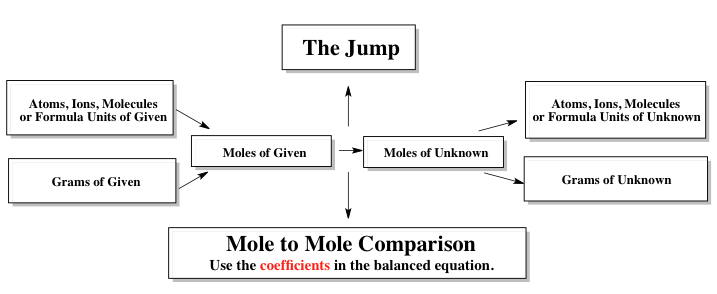Ch.1 - Chemical MeasurementsWorksheetSee all chapters
 Ch.1 - Chemical Measurements 2hrs & 10mins 0% complete Worksheet Ch.2 - Tools of the Trade 1hr & 26mins 0% complete Worksheet Ch.3 - Experimental Error 1hr & 51mins 0% complete Worksheet Ch.4 + 5 - Statistics, Quality Assurance and Calibration Methods 2hrs & 1min 0% complete Worksheet Ch.6 - Chemical Equilibrium 3hrs & 43mins 0% complete Worksheet Ch.7 - Activity and the Systematic Treatment of Equilibrium 1hr & 1min 0% complete Worksheet Ch.8 - Monoprotic Acid-Base Equilibria 1hr & 52mins 0% complete Worksheet Ch.9 - Polyprotic Acid-Base Equilibria 2hrs & 16mins 0% complete Worksheet Ch.10 - Acid-Base Titrations 2hrs & 22mins 0% complete Worksheet Ch.11 - EDTA Titrations 1hr & 37mins 0% complete Worksheet Ch.12 - Advanced Topics in Equilibrium 1hr & 18mins 0% complete Worksheet Ch.13 - Fundamentals of Electrochemistry 2hrs & 27mins 0% complete Worksheet Ch.14 - Electrodes and Potentiometry 55mins 0% complete Worksheet Ch.15 - Redox Titrations 1hr & 14mins 0% complete Worksheet Ch.16 - Electroanalytical Techniques 1hr & 23mins 0% complete Worksheet Ch.17 - Fundamentals of Spectrophotometry 55mins 0% complete Worksheet BONUS: Chemical Kinetics Not available yet

Volumetric Titrations

See all sections
Sections
SI Units
Metric Prefixes
Chemical Concentrations
Volumetric Analysis
Volumetric Titrations

Volumetric Calculations involves the use of both gravimetric analysis and stoichiometry

Titrations

Example #1: When dealing with volumetric calculations we utilize a stoichiometric chartExample #2: Iron (III) can be oxidized by an acidic K2Cr2O7 solution according to the net ionic equation below. How many microliters of a 0.250 M FeCl2 are needed to completely react with 9.12 g of a compound containing 41.5% weight K2Cr2O7?

Cr2O72-  +  6 Fe2+  + 14 H+   →   2 Cr3+  +  6 Fe3+   +  7 H2O

Titrations Calculations

Example #3: Magnesium reacts with HCl according to the reaction below. How many grams of 5.310% by weight of aqueous magnesium are required to provide a 25% excess to react with 75.0 mL of 0.0550 M HCl.

Mg (s)  +   2 HCl (aq)  →  MgCl2 (aq) +  H2 (g)

Example #4: The amount of iron within an ore sample was determined by an oxidation-reduction titration using potassium permanganate, KMnO­4, as the titrant. A 0.5600 g sample of the ore was placed into acid and the newly freed Fe3+ was then reduced to Fe2+. The titration of this solution required 39.82 mL of 0.0315 M KMnO­4 to reach the end-point. Determine the mass percent of Fe2O3 in the sample.

MnO4   +  5 Fe2+   + 8 H+  ⇌  Mn2+  +  5 Fe3+  +  4 H2O

Example #5: A 0.4317 g sample of CaCO3 (MW: 100.09 g/mol) is added to flask that also contained 12.50 mL of 1.530 M HBr.

CaCO3 (aq)  +  2 HBr (aq)  →  CaBr2 (aq)  +  H2O (l)  +  CO2 (g)

Additional water is then added to create a 250.0 mL of Solution A. Next 20.00 mL aliquot of solution A is taken and titrated with 0.0980 M NaOH. How many milliliters of NaOH were used?

NaOH (aq)  +  HBr (aq)  →  H2O (l)  +  NaBr (aq)

Example #6: A 9.2476 g sample of M(OH)2 was mixed with 15.00 mL of 1.530 M HI and diluted to a final 125.0 mL of solution.

M(OH)2 (aq)  +  2 HI (aq)  →  2 H2O (l)  +  MCl2 (aq)

A 12.00 mL aliquot of this diluted solution was taken and titrated with 18.23 mL of 0.0695 M NaOH.

NaOH (aq)   +  HI (aq)  → H2O (l)  +  NaCl (aq)

What is the identity of the metal representing M?

Example #7: A 6.2034 g sample of Cu(OH)n was mixed with 25.00 mL of 2.250 M Hl and diluted to a final 250.0 mL of solution.

Cu(OH)n (s)  +  n HI (aq)  →  CuIn (aq)  +  n H2O (l)

A 50.00 mL aliquot of this solution was taken and titrated with 16.25 mL of 0.1250 M KOH. What is the value of n?

KOH (aq)  +  HI (aq)  →  H2O (l)  +  NaI (aq)

Practice: A 1.000 g sample of Na2CO3 (MW: 105.99 g/mol) is dissolved in enough water to make 200.0 mL of solution. A 25.00 mL aliquot required 32.18 mL of HCl to completely neutralize it. What is the molar concentration of HCl?

Na2CO3 (aq)  + 2 HCl (aq)  →  2 KCl (aq)  +  H2O(l)  +  CO2  (g)